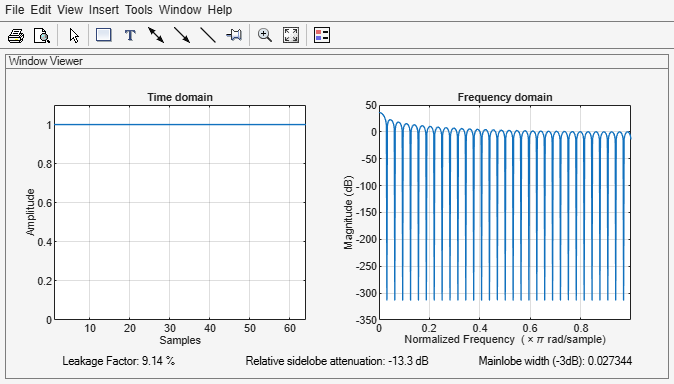Documentation

# rectwin

Rectangular window

## Syntax

``w = rectwin(L)``

## Description

example

````w = rectwin(L)` returns a rectangular window of length `L`.```

## Examples

collapse all

Create a 64-point rectangular window. Display the result using `wvtool`.

```L = 64; wvtool(rectwin(L))```## Input Arguments

collapse all

Window length, specified as a positive integer.

Data Types: `single` | `double`

## Output Arguments

collapse all

Rectangular window, returned as a column vector.

## Algorithms

The output of the `rectwin` function with input `L` can also be created using the `ones` function.

```w = ones(L,1); ```

 Oppenheim, Alan V., Ronald W. Schafer, and John R. Buck. Discrete-Time Signal Processing. Upper Saddle River, NJ: Prentice Hall, 1999, pp. 468–471.